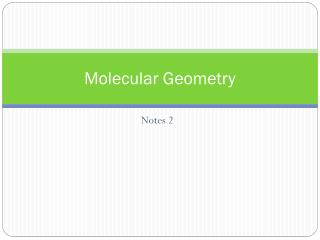# Molecular Geometry - PowerPoint PPT PresentationDownload PresentationMolecular Geometry

Molecular GeometryDownload Presentation## Molecular Geometry

- - - - - - - - - - - - - - - - - - - - - - - - - - - E N D - - - - - - - - - - - - - - - - - - - - - - - - - - -
##### Presentation Transcript

1. Molecular Geometry Notes 2

2. Molecules with Expanded Valence Shells • Atoms that have expanded octets have AB5 (trigonal bi- pyramidal or AB6 octahedral, electron domain geometries. • Trigonal bipyramidal structures have a plane containing three electron pairs • In this structure two trigonal pyramids share a base • For Octahedral structures, there is a plane containing four electron pairs • The fifth and sixth pair are located above and below this plane • Two square pyramids share a base.

3. Trigonal Bipyramid • The three electron pairs in the plan are called equatorial • The two electron pairs above and below the plane are called axial • The axial electron pairs are 180o apart and 90o from the equatorial sections • The equatorial pairs are 120o apart • To minimize electron- electron repulsions, nonbonding pairs are always placed in an equatorial position, and bonding pairs in either axial or equatorial positions.

4. Octahedral • Four electron pairs in the plane are at 90o to each other. • The remaining two electron pairs are 180o apart and 90o from the electrons in the equatorial plane. • Because of the symmetry of the system each position is equivalent • IF we have five bonding pairs and one nonbonding pair, it doesn’t matter where the nonbonding pair is placed • The molecular geometry is square pyramidal • IF two nonbonding pairs are present, the repulsions are minimized by pointing them toward opposite sides of the octahedron. • The geometry is square planar.

5. Molecules with more than one Central atom. • In acetic acid CH3COOH there are three central atoms two C and one O • We assign the molecular ( and electron domain ) geometry about each central atom sseparately: • The geometry around the first C is tetrahedral • The geometry around the second C is trigonal planar • The geometry around the O is tetrahedral

6. Polarity of Polyatomic Molecules • Polar molecules interact with electric fields • Binary compounds are polar if their centers of negative and positive charge do not coincide • If two charges, equal in magnitude and opposite in sign, are separated by a distance d, then a dipole is established • The dipole moment, m is given by: • m=Qr Where Q is the magnitude of the charge • We can extend this to polyatomic molecules

7. For each bond in a polyatomic molecule, we can consdier the bond dipole. • The dipole moment due only to the two atoms in the bond is the bond dipole. • Because bond dipoles and dipole moments are vector quanties, the orientation of the individual dipole moments determines whether the molecule has an overall dipole moment. • Example in CO2 each C-O bond is cancelled because the molecule is linear, but in H2O the dipoles do not cancel out because the molecule is bent

8. It is possible for a molecule with polar bonds to be either polar or non-polar depending on the symmetry. • For diatomic molecules AB • Polar bonds always result in an overall dipole moment • For triatomic molecules AB2 • If the molecule is bent there is an overall dipole moment • If the geometry is linear and both B atoms are the same, there is no overall dipole moment • If the geometry is linear and the B atoms are different, there is an overall dipole moment

9. For molecules with four atoms AB3 • If the geometry is trigonal pyramidal there is an overall dipole moment • If the molecular geometry is trigonal planar and B atoms are identical, there is no overall dipole moment • If the molecular geometry is trigonal planar and the B atoms are different, there is an overall dipole moment • If the geometry is T-shaped there is an overall dipole moment